### Home > PC > Chapter 6 > Lesson 6.2.1 > Problem6-74

6-74.
1. Christina stared at the equation tan x =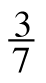. “That’s interesting,” she thought. “Since tan x =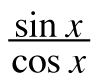, this must mean that sin x = 3 and cos x = 7. But that’s impossible.” Homework Help ✎

1. Why is it impossible?

2. What’s going on here?

3. Find x if 0 ≤ x ≤ 2π.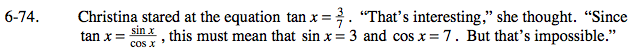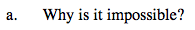What is the range of sine and cosine?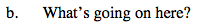$\text{For example, }\frac{0.3}{0.7}=\frac{3}{7}.$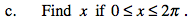$\text{Using a calculator evaluate }\tan^{-1}\left (\frac{3}{7} \right ).$

There is another solution. Use the unit circle to help you calculate the second solution.